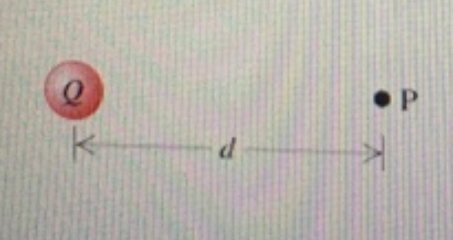# Problem: If the total positive charge is Q= 1.62×10-6 C, and the magnitude of the electric field caused by this charge at point P is 6220 N/C at a distance d= 1.53m from the charge. Now find the magnitude of the force on an electron placed at point P. Recall that the charge on an electron has magnitude e=1.60x10-19 C .Enter your answer numerically in newtons.

###### FREE Expert Solution

The electric force on a charged object is expressed as:

$\overline{){\mathbf{F}}{\mathbf{=}}{\mathbf{q}}{\mathbf{E}}}$, where q is charge and E is the electric field.

We've been given q = e = 1.60×10-19 C

97% (391 ratings)###### Problem Details

If the total positive charge is Q= 1.62×10-6 C, and the magnitude of the electric field caused by this charge at point P is 6220 N/C at a distance d= 1.53m from the charge. Now find the magnitude of the force on an electron placed at point P. Recall that the charge on an electron has magnitude e=1.60x10-19 C .